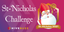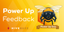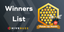# Advent of Functional PHP: Day 5

in PHPlast year

After the last two days, Day 5 of Advent of Code is almost mundane in comparison. Today we're asked to read in the definition for a series of lines and compute how many times they intersect.

The process is much the same as the previous days: Parse the incoming data into some sort of data model, then run some computations on it. And both parts will consist primarily of `pipe()` operations, since we're really just shuffling data from one form to another.

Our input data looks like this (albeit with a much larger range of coordinates):

``````0,9 -> 5,9
8,0 -> 0,8
9,4 -> 3,4
2,2 -> 2,1
7,0 -> 7,4
6,4 -> 2,0
0,9 -> 2,9
3,4 -> 1,4
0,0 -> 8,8
5,5 -> 8,2
``````

## Defining the data

Since we are dealing with a series of lines, and lines are defined by two points, that gives us a good idea of what the data model should be:

``````class Point
{
public function __construct(
) {}
}

class Line
{
public function __construct(
) {}
}
``````

There's only one more trivial class we'll need later, but we'll cross that bridge when we come to it.

## Parsing the input

We've seen this story before. Parsing the input means reading it in line by line, and converting each line to a Line object. We'll do it lazily, just for kicks:

``````/**
* @return Line[]
*/
function parseInput(string \$inputFile): iterable
{
return pipe(\$inputFile,
lines(...),
itmap(makeLine(...)),
);
}
``````

The real work is in the `makeLine()` function.

``````function makeLine(string \$line): Line
{
return pipe(\$line,
explode('->'),
amap(trim(...)),
amap(explode(',')),
amap(makePoint(...)),
static fn (array \$points): Line => new Line(\$points, \$points),
);
}
``````

By now, that should be all pretty boring. For each line, split it at the arrow, clean up whitespace, and then explode it again on a comma. That gives us a 2 element array of 2 element arrays: Two points, each of which is two numeric strings. We then map that first element array through `makePoint()`, that is, call makePoint() on each of the two points.

``````function makePoint(array \$coords): Point
{
return new Point((int)\$coords, (int)\$coords);
}
``````

`makePoint()` takes the 2-element array of numeric strings that is a single point, coerces them to real ints, and makes a `Point`. Now that we have an array that consists of two `Points` instead of two arrays, we pass that array to an anonymous function that does the exact same thing to make a `Line`.

Note that there's really no difference between `makePoint()` being a named function or an anonymous function. We could also have made it a static method on the `Point` class if we wanted to. The only reason I made the line-maker an anonymous function is `makeLine()` was already the name of a function, and I didn't feel like making a new name. All of these would work fine. The only thing you can't do is call the constructor as a piped function, because the `func(...)` syntax doesn't work on constructors. (It works on named constructors, aka static methods, though, if you are so inclined.)

That was easy enough, aside from the slight trickery of exploding the data twice. The result is nice and compact, though.

## Find the overlaps

For part 1, we need only consider those lines that are exactly horizontal or vertical. That's a strong hint we will need a filter operation, and a function to determine if a line is orthogonal or not. We could make that a method on the `Line` class, but... meh. In functional code, methods frequently don't offer much benefit, and in fact become harder to compose. The `pipe()` operation can't syntactically handle "call this method on the value that is passed in," because the value passed in has no variable. (This style is often called "point-free," meaning "without interstitial variables, or points.")

In any case, here's our function:

``````function isOrthogonal(Line \$line): bool
{
return (\$line->start->x === \$line->end->x)
|| (\$line->start->y === \$line->end->y);
}
``````

We don't actually care if it's horizontal or vertical, just if it's one of them, so it returns true for either case.

Now that we have just the data we care about, we run into the challenge of math. (Not to be confused with arithmetic. Math is fun. I got into programming so that I wouldn't have to do arithmetic. That's also why I rarely do anything fancy in CSS, but I digress.) How do we tell if two lines intersect? If we had lines as mathematical formulae there are algebraic ways to do so, but we don't.

The next thought is to materialize the lines into an array of points, and then check for any point that appears in more than one array. That would work, but would be extraordinarily slow. It would require doing an `array_intersect()` call between every two combinations of lines, meaning it's an O(n!) algorithm. (That's "n factorial", also known as O(crap).) So that's no good.

What we can do instead is materialize all of the lines onto a common grid. At each grid cell, increment a counter for each point in a line. If any grid cells end up with a counter of 2 or more, there's an intersection. That's still not an especially fast algorithm, but it's the best we can easily do.

"Go over a list of points and add them to a grid" should sound an awful lot like the `reduce()` operation we've become so friendly with in the past few days. Because PHP passes arrays by value, we technically could just pass an array through the reduce operation and modify it "in place" in the function. That would work, and still be functional since the reducing function itself is pure. I personally prefer to keep my data in objects, though, even if they're trivial objects, so we'll make a trivial `Grid` class. This one is personal preference, and it's my blog post so I get to decide.

``````class Grid
{
public function __construct(
public readonly array \$grid = [],
) {}
}
``````

Now we need our reducing function, which takes a grid and a line. Well, not a line, actually. It takes a list of points that a line produces. That means we first need to materialize a line into an array of `Point`s, and then reduce over the list of those point arrays.

``````/**
* @return Point[]
*/
function materializeLine(Line \$line): iterable
{
foreach (range(\$line->start->x, \$line->end->x) as \$x) {
foreach (range(\$line->start->y, \$line->end->y) as \$y) {
yield new Point(\$x, \$y);
}
}
}
``````

Here we see our first use of a non-generic generator. Because we know the lines are either vertical or horizontal, we can iterate over both axes and `yield` a new `Point` for each combination. One or the other `foreach()` loop is guaranteed to have only a single iteration, and for our purposes we don't really care which it is. The result is a generator that will lazily produce `Point` objects as needed.

We can then `reduce()` over that generator (because our `reduce()`, unlike PHP's built-in one, can work on any iterable) with our reducing function. That function is quite simple:

``````function markLine(Grid \$old, iterable \$points): Grid
{
\$grid = \$old->grid;

foreach (\$points as \$point) {
\$grid[\$point->x][\$point->y] = (\$grid[\$point->x][\$point->y] ?? 0) + 1;
}

return new Grid(\$grid);
}
``````

For each point, we increment the number stored at a given coordinate. The quirky syntax is because we're not pre-initializing the grid to 0; a 0 is represented by simply not existing, so we default not-existing to 0, and add 1. Also note this means we are not storing a value at every coordinate! There will only be data at coordinates that have at least one point. Given that the input data has a range of just under 1000x1000, that's a non-trivial memory savings.

Finally, we have a `Grid` that contains all of the lines marked onto it. Now we just need to find any overlaps, that is, any cells with a value of 2 or more. The naive way would be to double-iterate the grid and increment a counter for any number greater than 1. But we can be more functional than that.

Recall from previous days that we have a `flatten()` function available that flattens a 2D array into a single list. We don't care about coordinates anymore, just values, so that simplifies the process a bit. Then we have a list of marks, and we want the count of elements where the value is >1. OK, so filter by our criteria and count the result.

``````function countOverlaps(Grid \$grid): int
{
return pipe(\$grid->grid,
flatten(...),
afilter(static fn (int \$cell): bool => \$cell > 1),
count(...),
);
}
``````

We now have all of our necessary steps. And with their powers combined, we have a pipe!

``````\$in = parseInput(\$inputFile);

\$overlaps = pipe(\$in,
itfilter(isOrthogonal(...)),
itmap(materializeLine(...)),
reduce(new Grid, markLine(...)),
countOverlaps(...),
);
``````

## Diagon Alley

Part 2 wants us to consider all the lines, not just the horizontal and vertical ones. Fortunately, we're also told that the remaining lines are guaranteed to be perfectly diagonal, which makes computing their materialized points a lot easier.

There's only two changes needed to our pipeline. One, we can remove the `isOrthogonal()` filter as we no longer want to filter the list. However, we'll still need that function inside `materializeLine()`.

Specifically, materializing an orthogonal line and a diagonal line require different steps. There's only those two options, so let's just fork the function:

``````function materializeLine(Line \$line): iterable
{
yield from isOrthogonal(\$line)
? materializeOrthogonalLine(\$line)
: materializeDiagonalLine(\$line);
}
``````

`materializeOrthogonalLine()` is the exact logic we saw before. `materializeDiagonalLine()` gives us the `Point`s for a diagonal line. For that, we can take advantage of the fact that the there will be an equal number of steps between the start and end x values and the start and end y values. So if we pair up the first x with first y, second x with second y, etc., we'll get our points.

That "pair up" could be done with `array_combine()` if we wanted to use the array key, but for fun we'll instead use a `zip()` operation. A `zip()` takes two arrays and produces a single array of pairs, one from each array. PHP doesn't have a native `zip()`, but the native `array_map()` defaults to becoming one if a `null` callback is passed. (From the Weird But True(tm) department.) That means we can `zip()` the two arrays, then map those points to the `Point` constructor.

``````/**
* @return Point[]
*/
function materializeDiagonalLine(Line \$line): iterable
{
\$xrange = range(\$line->start->x, \$line->end->x);
\$yrange = range(\$line->start->y, \$line->end->y);

return pipe(array_map(null, \$xrange, \$yrange),
itmap(static fn (array \$pair) => new Point(...\$pair))
);
}
``````

Conveniently, `\$pair` is already in the right order to pass into the `Point` constructor so we can just splat it. (The `...` variadic operator is known as `splat`. No, the documentation doesn't say that, but that's what it's called, and that's what I will always call it. Don't @ me.)

With those two changes, our job here is done.

## It's pipes all the way down

A fun bit to mention here is that as long as all of our functions are pure (which they are), function composition is associative. That is, it doesn't matter where you put the parentheses. Put another way, all of the named functions above that consist just of a pipe can be optimized away into one long pipe command. The one caveat is the mapping over `makeLine()`, but we can inline a `compose()` command for that.

What that all means is that we could, if we wanted to, rewrite the above like so:

``````\$overlaps = pipe(\$inputFile,
lines(...),
itmap(compose(explode('->'),
amap(trim(...)),
amap(explode(',')),
amap(makePoint(...)),
static fn (array \$points): Line => new Line(\$points, \$points),
)),
itmap(materializeLine(...)),
reduce(new Grid, markLine(...)),
static fn (Grid \$g): array => \$g->grid,
flatten(...),
afilter(static fn (int \$cell): bool => \$cell > 1),
count(...),
);
``````

That's it. That's the program. You can read the entire flow of the program right there in one spot. The one caveat is `materializeLine()`, because it has internal logic that is more than just a `pipe()`. If we really really wanted to, though, we could develop a utility function that handles the branching between the true and false case, which would let us inline both `materializeLine()` and `materializeDiagonalLine()`. With a little rewriting we could probably rewrite `materializeOrthogonalLine()` as a pipe, too, and inline that.

I'm not convinced it would be wise to go that far in inlining things, but it's cool that we could. That also means we can break up the flow into sub-functions anywhere we want. Any segment of that pipe can be pulled out to a named function and then called as a pipe step, depending on what we decide would be most readable and reusable.

That is the power of functional composition.

As usual, the code is on GitHub. See you back here for the next one.

Want to know more about functional programming and PHP? Read the whole book on the topic: Thinking Functionally in PHP.Congratulations @crell! You have completed the following achievement on the Hive blockchain and have been rewarded with new badge(s):You received more than 5000 upvotes.Your next target is to reach 6000 upvotes.

If you no longer want to receive notifications, reply to this comment with the word `STOP`Saint-Nicholas challenge for well-behaved girls and boysFeedback from the December 1st Hive Power Up DayHive Power Up Month Challenge - Winners List